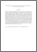# Relationship between mathematical thinking, mathematics anxiety and mathematics attitudes among university students

## Citation

Kargar, Maryam and Ahmad Tarmizi, Rohani and Bayat, Sahar (2010) Relationship between mathematical thinking, mathematics anxiety and mathematics attitudes among university students. Procedia - Social and Behavioral Sciences, 8. pp. 537-542. ISSN 1877-0428

## Abstract

This study investigates the relationships between mathematics anxiety, attitudes toward mathematics and mathematical thinking among university students. A 60-item questionnaire included mathematical thinking rating scale, mathematics anxiety rating scale and mathematics attitudes rating scale completed by 203 university students from the Faculty of Science, Engineering, Food Science, and Human Ecology in one of the public university in Malaysia. The correlation analysis was used to establish relationship between mathematical thinking, mathematics attitude, and mathematics anxiety and independent t-test was used to investigate differences between the two gender groups and two race groups on their mathematics anxiety, attitudes toward mathematics and mathematical thinking. The results indicated that a significant high positive correlation exists between mathematical thinking and mathematics attitude (r=.856, p<0.05). Also there was negative moderate correlation between mathematical thinking and mathematics anxiety (r=.-576, p<0.05). There was also negative correlation between mathematics anxiety and mathematics attitude (r=.509, p<0.05). In conclusion, these findings indicated that level of mathematics anxiety is related to mathematical thinking and mathematics attitude. On the other hand, students' backgrounds in terms of their majors have no effect on the three constructs.Preview
PDF (Abstract)
Relationship between mathematical thinking.pdfView Item